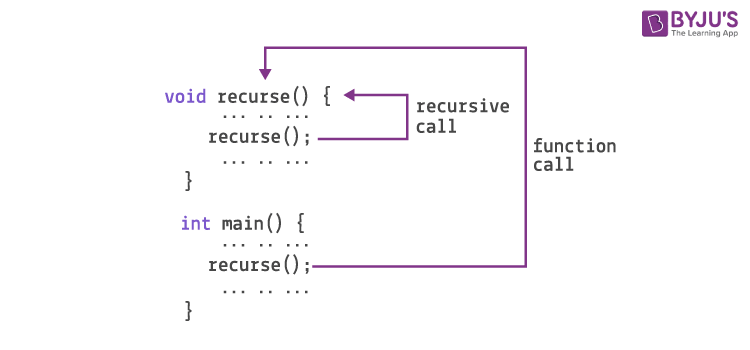# Introduction to Recursion | GATE CSE Notes

Recursion is an important topic from the Computer Science family. And, when it is about competitive examinations like GATE, you need to know each aspect of recursion. This article will help you understand the recursion topic in a better way.

## What is Recursion?

When in any program a function calls itself, it is called recursion. It can happen directly or indirectly. The method of call leads to different types of recursion. Recursion is a problem-solving technique that contains some special properties. In the process of recursion, the function breaks down into different parts to solve the problem.## Types of Recursion

There are two types of Recursion.

1. Direct Recursion
2. Indirect Recursion

1. Direct Recursion: When we need to call just a single function by itself, direct recursion is used. It is an easier way that includes a single step to call the original function or method.

1. Indirect Recursion: When we call more than one function through another function, it is called indirect recursion. Such a calling method can be implemented multiple times.

## Conditions to use Recursion

1. Recursion is very suitable for data abstraction.
2. It is best to use recursion when there are multiple operations to be implemented on a single data.
3. Recursion helps to easily read and maintain large data.
4. If the problem you are finding a solution for is mentioned in the recursive term, then recursion can be used.
5. Recursion simplifies the implementation of algorithms, thus it can be used in such situations.

1. Recursion helps to reduce the complexity in any program. Its implementation is simple, as you just need to define the base condition and recursion case in the recursive function.
2. Recursion is a time-saving method. It reduces the time required to write or debug the program.
3. Recursion is the most simplified way for tree traversal.

1. Recursion consumes memory.
2. If not implemented correctly, recursion can be time-consuming.

## Practice Problem- Recursion

Q. Consider the following C program:

#include <stdio.h>

int counter = 0;

int calc (int a, int b) {

int c;

counter++;

if (b==3) return (a*a*a);

else {

c = calc(a, b/3);

return (c*c*c);

}

}

int main (){

calc(4, 81);

printf (“%d”, counter);

}

The output of this program is _____.

Q. Consider the following program written in pseudo-code. Assume that x and y are integers.

Count(x,y) {

if (y != 1){

if (x != 1) {

print(“*”);

Count(x/2, y);

}

else {

y = y-1;

Count(1024, y);

}

}

}

The number of times that the print statement is executed by the call Count(1024,1024) is _____.

## Video on RecursionKeep learning and stay tuned to get the latest updates on GATE Exam along with GATE Eligibility CriteriaGATE 2023GATE Admit CardGATE Syllabus for CSE (Computer Science Engineering)GATE CSE NotesGATE CSE Question Paper, and more.

Also Explore,